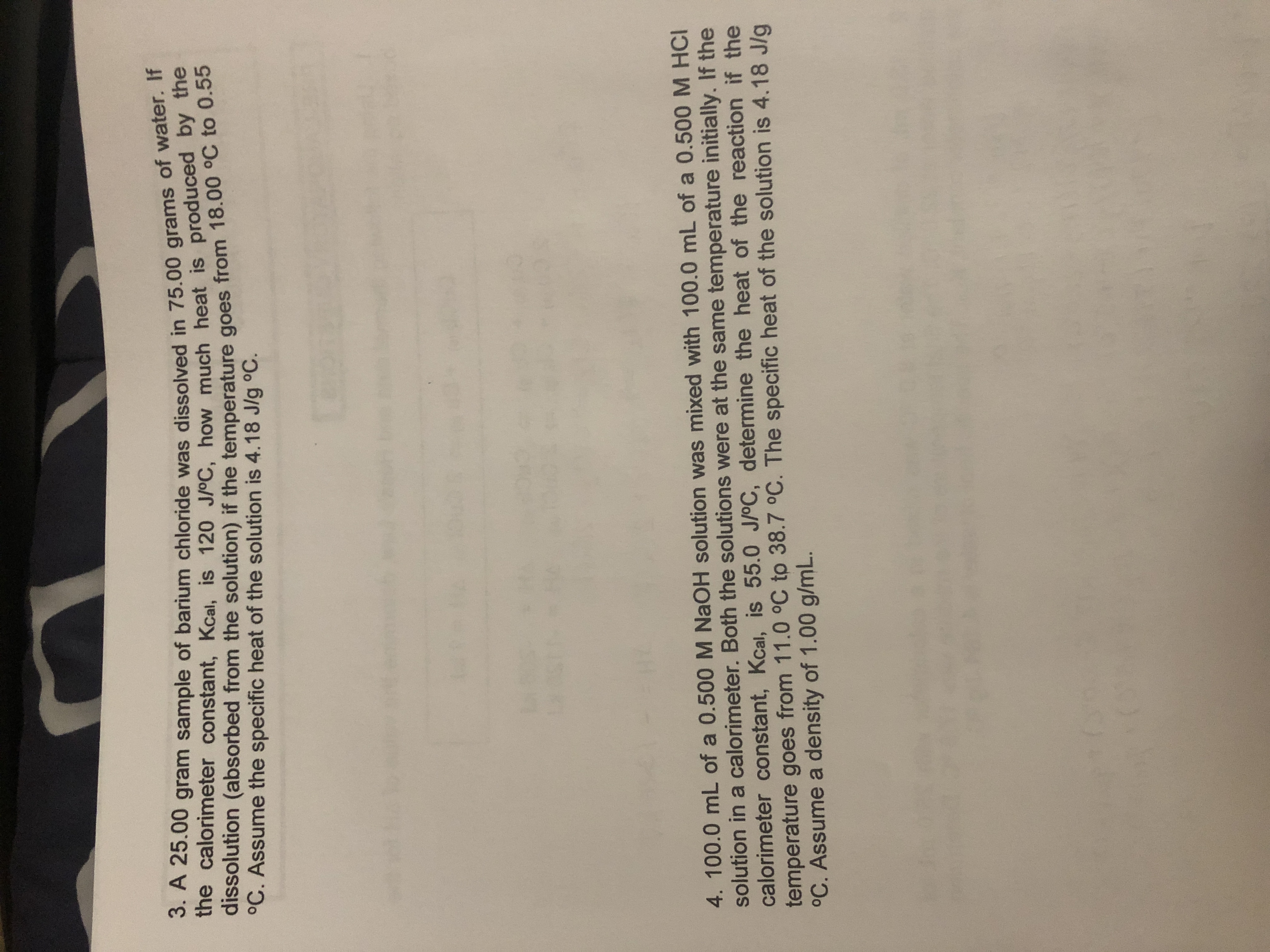# 3. A 25.00 gram sample of barium chloride was dissolved in 75.00 grams of water. If the calorimeter constant, Kcal, is 120 J/oC, how much heat is produced by the dissolution (absorbed from the solution) if the temperature goes from 18.00 °C to 0.55 °C. Assume the specific heat of the solution is 4.18 J/g °C. 4. 100.0 mL of a 0.500 M NAOH solution was mixed with 100.0 mL of a 0.500 M HCI solution in a calorimeter. Both the solutions were at the same temperature initially. If the calorimeter constant, Kcal, is 55.0 J/oC, determine the heat of the reaction if the temperature goes from 11.0 °C to 38.7 °C. The specific heat of the solution is 4.18 J/g °C. Assume a density of 1.00 g/mL.

Question

Question 3help_outlineImage Transcriptionclose3. A 25.00 gram sample of barium chloride was dissolved in 75.00 grams of water. If the calorimeter constant, Kcal, is 120 J/oC, how much heat is produced by the dissolution (absorbed from the solution) if the temperature goes from 18.00 °C to 0.55 °C. Assume the specific heat of the solution is 4.18 J/g °C. 4. 100.0 mL of a 0.500 M NAOH solution was mixed with 100.0 mL of a 0.500 M HCI solution in a calorimeter. Both the solutions were at the same temperature initially. If the calorimeter constant, Kcal, is 55.0 J/oC, determine the heat of the reaction if the temperature goes from 11.0 °C to 38.7 °C. The specific heat of the solution is 4.18 J/g °C. Assume a density of 1.00 g/mL. fullscreen